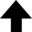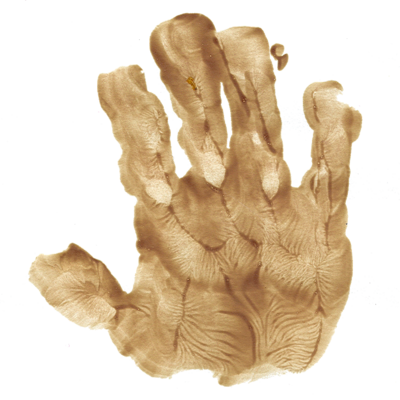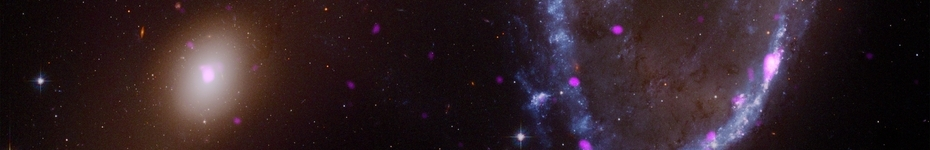You must come to appreciate that if QED can explain the interaction of charged particles, then it can effectively explain all of fundamental chemistry, since all of chemistry involves the interaction of electrons.Richard Feynman

## Attenuation of Photons in Matter

Unlike energetic charged particles which have a finite range when traversing matter, photons of a given energy do not possess such a discrete range, but rather are absorbed exponentially as a function of distance in the absorbing medium. This phenomenon is referred to as attenuation. For a beam of $n_0$ monoenergetic photons, the rate of absorption of the photons as a function of depth in the absorber is

 $\displaystyle{ -\frac{\mathrm{d} n}{\mathrm{d} x} = N \sigma n_0 \text{,} }$ (

where $\sigma$ is the absorption cross section of each atomic scattering center (or absorbing molecule), and $N$ is the volume density of atomic scattering centers. (The negative sign above represents absorption.) We define $\mu \equiv N \sigma$, where $\mu$ is referred to as the linear attenuation coefficient. We can then integrate Equation (1) with respect to distance $x$ to obtain

 $\displaystyle{ n(x) = n_0 \mathrm{e}^{-\mu x} \text{.} }$ (

Similarly, if we consider depth $x$ in terms of not distance, but of areal density (in g/cm2), we can define the mass attenuation coefficient such that

 $\displaystyle{ \mu_\text{m} \equiv \frac{\mu}{\rho} = \frac{N \sigma}{\rho} = \frac{N_\text{A} \sigma}{\bar{A}} \text{,} }$ (

where $\rho$ is the mass density (in g/cm3), $\bar{A}$ is the atomic molar mass (in g/mol) of the absorbing medium, and $N_\text{A}$ is Avogadro's number (in molecules/mol), respectively. Equation (2) now becomes:

 $\displaystyle{ n(x) = n_0 \mathrm{e}^{-\mu_\text{m} x} \text{.} }$ (

Practical physics applications typically make use of Equation (4) wherein values of $\mu_\text{m}$ are tabulated.

## Mechanisms of Photon Interaction with Matter

There are a number of different mechanisms by which photons can interact with matter. However, only three of these mechanisms—the photoelectric effect, Compton scattering, and electron/positron pair production—dominate, while the remainder usually make only a negligible contribution. The total absorption cross section $\sigma$ in Equation (3) is the sum of the cross sections for each type of photon interaction:

 $\displaystyle{ \sigma = \sigma_\text{PE} + \sigma_\text{C} + \sigma_\text{PP} \text{,} }$ (

where $\sigma_\text{PE}$ is the cross section for photoelectric interactions, $\sigma_\text{C}$ is the cross section for Compton interactions, and $\sigma_\text{PP}$ is the cross section for pair production. (A cross section in nuclear physics can informally be read as an energy-dependent probability for interaction.) Since $\mu \propto \sigma$ from Equation (3), there is a corresponding mass attenuation coefficient for each cross section.

The cross sections given in Equations (6), (8), and (10) below are given in terms of the circular electron area $\pi r_\text{e}^2$ the photon "sees", scaled by a function that is dependent on the energy of the incident photon $E_\gamma$. In all cases $E_\gamma$ is compared to the rest mass-energy of the electron $m_\text{e} c^2$, suggesting a deep relationship between the two.

These cross sections are implemented in a Fortran program for a single photon. If you're not a fortranmann, then it is also here in JavaScript:

 Energy of gamma: MeV Target: Carbon Aluminum Titanium Copper Lead Polyethylene Water CR-39 Kapton Air Teflon Silicon dioxide PVC Aluminum oxide Nylon Photoelectric effect: cm2 Compton scattering: cm2 Pair production: cm2 Total cross section: cm2 Attenuation length: cm

## Contents

### Photoelectric Effect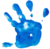Free electrons cannot fully absorb photons without violating conservation of energy and conservation of momentum, and for that reason, free electrons are not subject to the photoelectric effect. Indeed, the photoelectric effect should be thought of as interaction between an incident photon and an entire atom, not just one of its electrons.

Photoelectric interactions are more likely to eject electrons from the more tightly bound inner electron shells of the atom than the less tightly bound outer shells, since interactions involving inner shell electrons occur closer to the center of the atom where it is easier to transfer an incident photon's linear momentum to the atom. This is because nearly all the mass of the atom is concentrated in its nucleus.

The cross section for the photoelectric effect is:

 $\displaystyle{ \sigma_\text{PE} = 4 \sqrt{2} \alpha^4 \bar{Z}^5 \frac{8 \pi r_\text{e}^2}{3} \left( \frac{m_\text{e} c^2}{E_\gamma} \right)^{7/2} \text{,} }$ (

where $\alpha$ is the fine-structure constant, $\bar{Z}$ is the atomic number of the absorbing medium, $r_\text{e}$ is the classical electron radius, the quantity $m_\text{e} c^2$ is the rest mass-energy of the electron, and $E_\gamma$ is the energy of the incident photon. Equation (6) should be applied if E > 0.1_dp .AND. E < 0.35_dp, or where the photon energy is above the K-absorption edge. Please see the Notes for more details.

### Compton ScatteringThe interaction of photons with "nearly" free electrons is described by a number of mechanisms:

• Photon interactions with "truly" free electrons is referred to as Thomson scattering;
• Coherent (or elastic) photon interactions with "nearly" free electrons is referred to as Rayleigh scattering;
• Incoherent (or inelastic) photon interactions with "nearly" free electrons is referred to as Compton scattering.

Figure 1 illustrates Compton scattering, where we assume that the target electron is "free" (i.e., not bound to a host atom). An incident photon of energy $E_\gamma$ "collides" with a free electron at rest, transferring some of its energy and momentum to the electron. The photon, now with energy $E'_\gamma$, scatters at an angle $\theta$, while the electron recoils at an angle $\phi$ with velocity $v = \beta c$.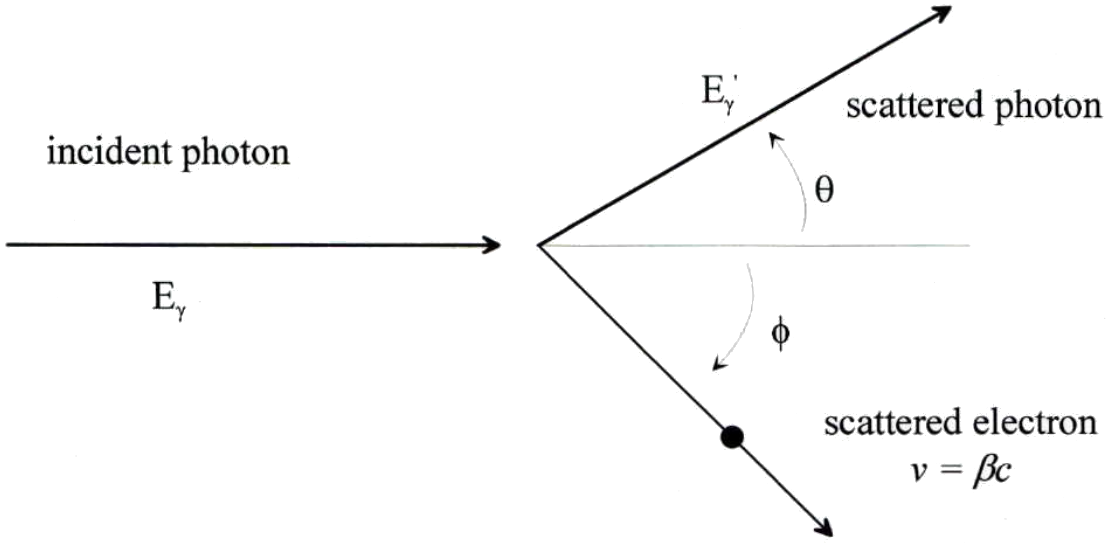Figure . The geometry of Compton scattering. Diagram courtesy of Eric Benton.

If we know the energy of the incident photon $E_\gamma$, and are able to experimentally detect the scattered photon and thereby determine the angle $\theta$, we can find the energy of the scattered photon $E'_\gamma$ by means of conservation of energy and conservation of momentum. Conservation of energy for the Compton scattering interaction illustrated in Figure 1 allows us to write

 $\displaystyle{ E_\gamma + m_\text{e} c^2 = E'_\gamma + \frac{m_\text{e} c^2}{\sqrt{1 - \beta^2}} \text{,} }$ (

where $\beta \equiv v /c = \sqrt{1 - \left[ m_\text{e} c^2 / (E_\gamma - E'_\gamma + m_\text{e} c^2) \right]^2 }$ from special relativity. After a lot of algebra, you will first write down the differential cross section for Compton scattering, which is specified by the Klein-Nishina formula. Integrating this formula over all angles gives the cross section for the Compton interaction:

 $\displaystyle{ \sigma_\text{C} = \frac{\pi r_\text{e}^2}{\bar{E}} f(\bar{E}) \text{,} }$ (

where the function $f(\bar{E})$ is equal to $\left( 1 - \frac{2 (\bar{E} + 1)}{\bar{E}^2} \right) \ln(2 \bar{E} + 1) + \frac{1}{2} + \frac{4}{\bar{E}} - \frac{1}{2 (2 \bar{E} + 1)^2}$. The factor $\bar{E}$ effectively compares the incident photon energy to the rest mass-energy of the electron, or $E_\gamma / m_\text{e} c^2$.

### Pair ProductionPair production is essentially the reverse process of bremsstrahlung. When in close proximity to a heavy nucleus, a photon can be converted into an electron/positron pair. Conservation of energy for this process dictates

 $\displaystyle{ E_\gamma = T_\text{e} + m_\text{e} c^2 + T_\text{p} + m_\text{e} c^2 \text{,} }$ (

where $T_\text{e}$ and $T_\text{p}$ are the kinetic energy of the electron and positron, respectively. Because the rest mass of the electron and the positron must come from the energy of the photon, there is a threshold energy of $2 m_\text{e} c^2$ = 1.022 MeV below which this process cannot occur.

The cross section for pair production originates from quantum electrodynamics, and is given by [R.J. Gould and G.P. Schréder, "Pair Production in Photon-Photon Collisions," Phys. Rev. 155, 1404 (1967)]:

 $\displaystyle{ \sigma_\text{PP} = \frac{\pi r_\text{e}^2}{2} (1 - \beta^2) f(\beta) \text{,} }$ (

where the function $f(\beta)$ is equal to $(3 - \beta^4) \ln \left( \frac{1 + \beta}{1 - \beta} \right) - 2 \beta (2 - \beta^2)$. As before, the factor $\beta \equiv v /c = \sqrt{1 - \left[ m_\text{e} c^2 / (E + m_\text{e} c^2) \right]^2 }$ is from special relativity. In this case $E$ is actually the relativistic kinetic energy of the electron/positron in the center-of-mass (CM) frame of reference.

#### Acknowledgement

I wish to thank Dr. Eric Benton for the nuclear and particle physics notes that were used as inspiration for this page.